CSE 463/563, Spring 2005

# Satisfaction of a Quantified WFF

 Last Update: 4 March 2005 Note: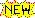ormaterial is highlighted

In case my explanation in lecture of the definition of satisfaction for a quantified wff wasn't clear, here it is at greater length (for a finite case, which may make it easier to understand, and can be done "wolog", i.e. "without loss of generality").

Suppose our domain,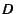, contains the following items: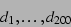Suppose our language has these variables:The definition of satisfaction for a universally quantified wff is: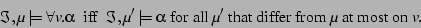E.g., supposeis the variable: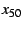and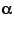is the wff: "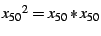"

Consider the set of all variables:Consider what set of members ofwe get by applying a variable assignment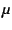to all the members of this set: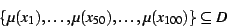Let's say that this set =.

Now consider all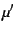that differ fromat most on. Suppose they are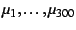.

(It may be that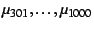differ fromon other variables, too, so we don't consider these at all!)

Note that ourabove is one of these 300(because all thediffer at most on, meaning that they might not differ onat all). For convenience, let's say that ourabove is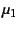.

So consider what all thesedo to the set of all variables; they map them into sets of elements ofas follows:Note, in particular, thatgives us:. What about the others? Well, each differs from(or) at most on what it does to, so we get the following:I.e., they are all alike on the first 49 variables and on the last 50 variables, but they can vary wildly on good old.

Now, according to the definition, if all of thesesatisfy "", then our originalwill satisfy "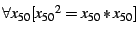".

But to decide if a givensatisfies "", we can ignore what it does to everything except.

(We can do this for 2 reasons: First, they don't differ on the other variables. Second, our wff only contains that one variable, so that's the only one we care about.)

On, eachdiffers. Does each one satisfy ""? Sure! Here's the proof: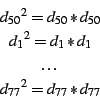So: all thethat differ from mu at most onsatisfy "", sosatisfies "".

And similarly, "mutatis mutandis" (as mathematicians say; i.e., changing what needs to be changed), for the existential quantifier.